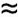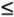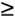# Chapter 14

[Page 787 ( continued )]
5. 9. 11. 13. 27. 29.
 1. (a and b)3.48, EV = 3.65, the results differ because not enough simulations were done in part a; (c) approximately 21 calls; no; repeat simulations to get enough observations 3. (a and b)2.95 sun visors 7. (a)\$256 (a) average time between arrivals4.3 days, average waiting time6.25 days, average number of tankers waiting= 1.16; (b) system has not reached steady state total yardage155 yd.; the sportswriter will predict Tech will win [ Tribune Daily News ] = [.50 .50]; too few iterations to approach a steady state 15. expansion is probably warranted 17. two of five trials (depended on random number stream) 19. system inadequate 21. avg. Salem dates = 2.92 23. P (capacity > demand) = .75 25. avg. maintenance cost = \$3,594.73; P (cost\$3,000) = .435 avg. rating = 2.91; P ( x3.0) = .531 (a) P (1,2) = .974, P (1,3) = .959, P (1,4) = .981, P (2,3) = .911, P (2,4) = .980, P (3,4) = .653; (b) (1,4) and (2,4). 32. (a) 700 rooms; (b) 690 roomsIntroduction to Management Science (10th Edition)
ISBN: 0136064361
EAN: 2147483647
Year: 2006
Pages: 358

Similar book on Amazon

flylib.com © 2008-2017.
If you may any questions please contact us: flylib@qtcs.net